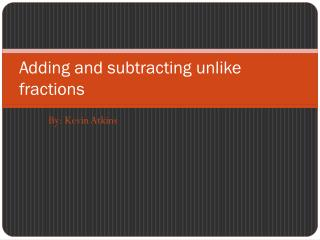DownloadDownload Presentation# Adding and subtracting unlike fractions

Télécharger la présentation## Adding and subtracting unlike fractions

- - - - - - - - - - - - - - - - - - - - - - - - - - - E N D - - - - - - - - - - - - - - - - - - - - - - - - - - -
##### Presentation Transcript

1. Adding and subtracting unlike fractions By: Kevin Atkins

2. Tutorial • 1.Line up denominators. • 2.See which denominator can go into it. • 3.Multiply to get the same denominator. • 4.What you do to the top you do to the bottom. • Now add and subtract your fractions.

3. Adding 10 unlike fractions 1.1/2+1/3= 2.2/3+1/4= 3.4/8+2/6= 4.8/8+3/3= 5.6/6+2/5= 6.7/9+3/5= 7.5/6+2/5= 8.7/7+3/4= 9.3/9+4/5= 10.3/7+4/7=

4. Subtracting10 unlike fractions 1.1/2-1/3= 2.2/3-1/4= 3.4/8-2/6= 4.8/8-3/3= 5.6/6-2/5= 6.7/9-3/5= 7.5/6-2/5= 8.7/7-3/4= 9.3/9-4/5= 10.3/7-4/7=